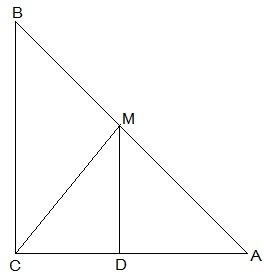# ABC is a triangle right angled at C. A line through the mid-point M of hypotenuse AB and parallel to BC intersects AC at D. Show that (i) D is the mid-point of AC (ii) MD ⊥ AC (iii) CM = MA = 1/2 AB(i) D is the mid-point of AC

Considering ∆ABC

We observe that M is the mid point of side AB and DM || BC [Given]

This implies, DC= AD ……….. (NCERT Theorem 8.10)

Hence, D is the mid-point of AC.

(ii) MD ⊥ AC

We know that MD || BC and AC is transversal

This implies, ∠ACB = ∠ADM = 90°

Hence, MD ⊥AC

(iii) CM = MA = ½ AB

AD = CD (D is the mid point of AC (Proved))

∠CDM = ∠ADM (proved, MD ⊥AC)

DM = DM (common)

∆ADM ≅ ∆CDM (By SAS congruency)

CM = AM (By C.P.C.T.)

CM = AM = ½ AB (M is the mid point of AB)(43)(13)